Examples

Chapter 6 Class 12 Application of Derivatives
Serial order wise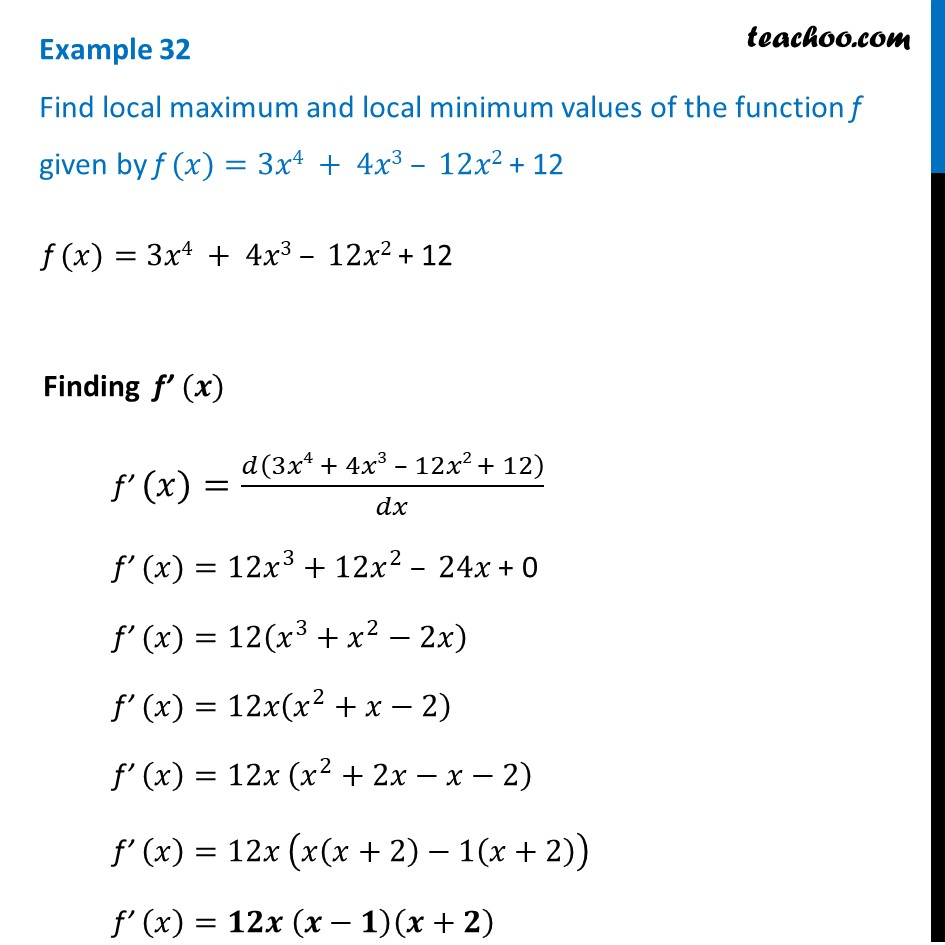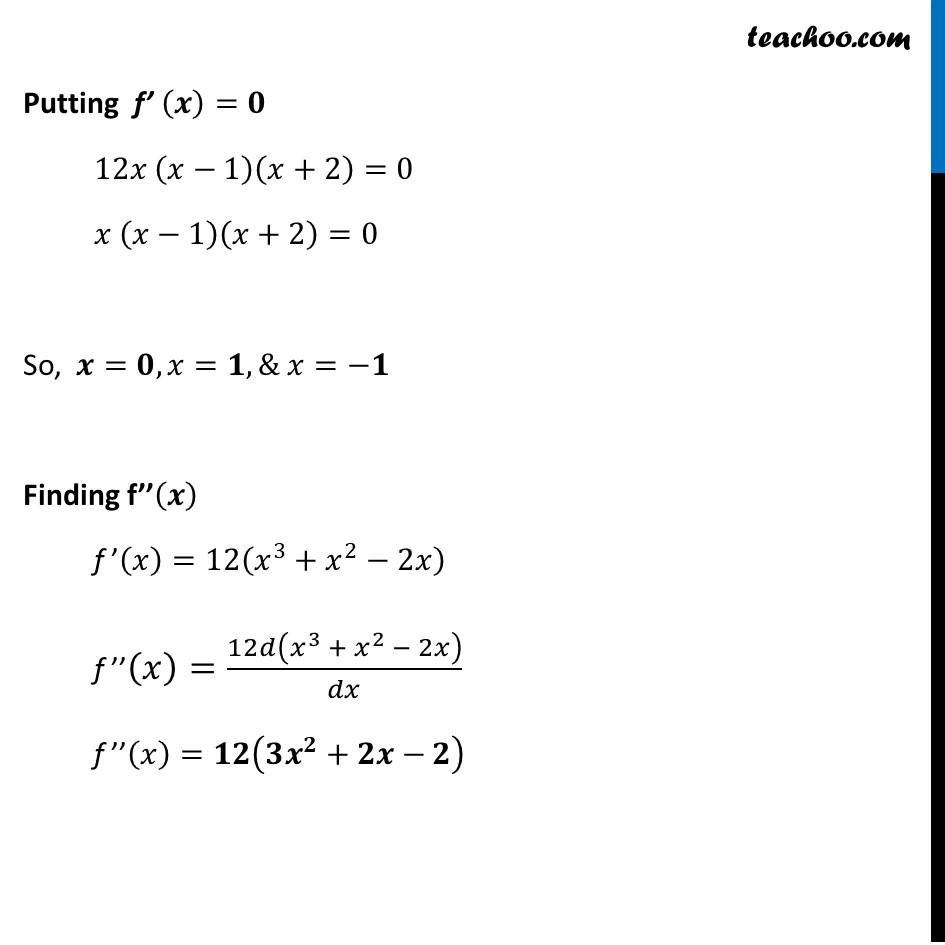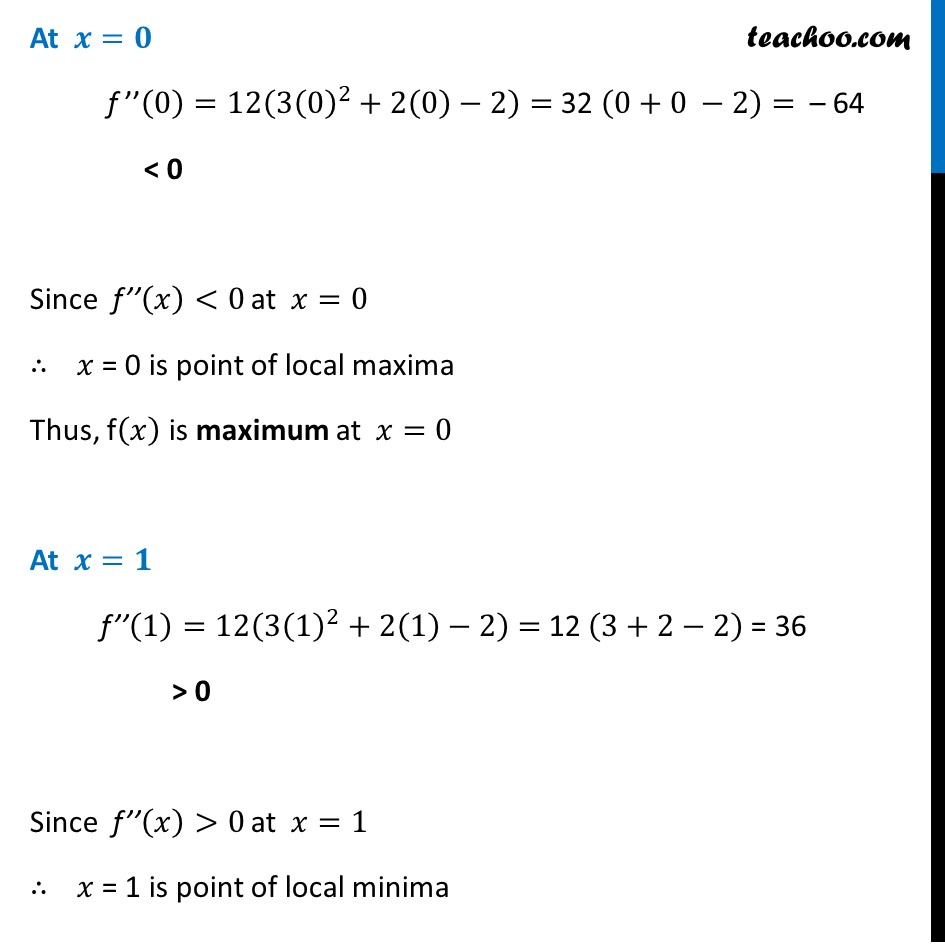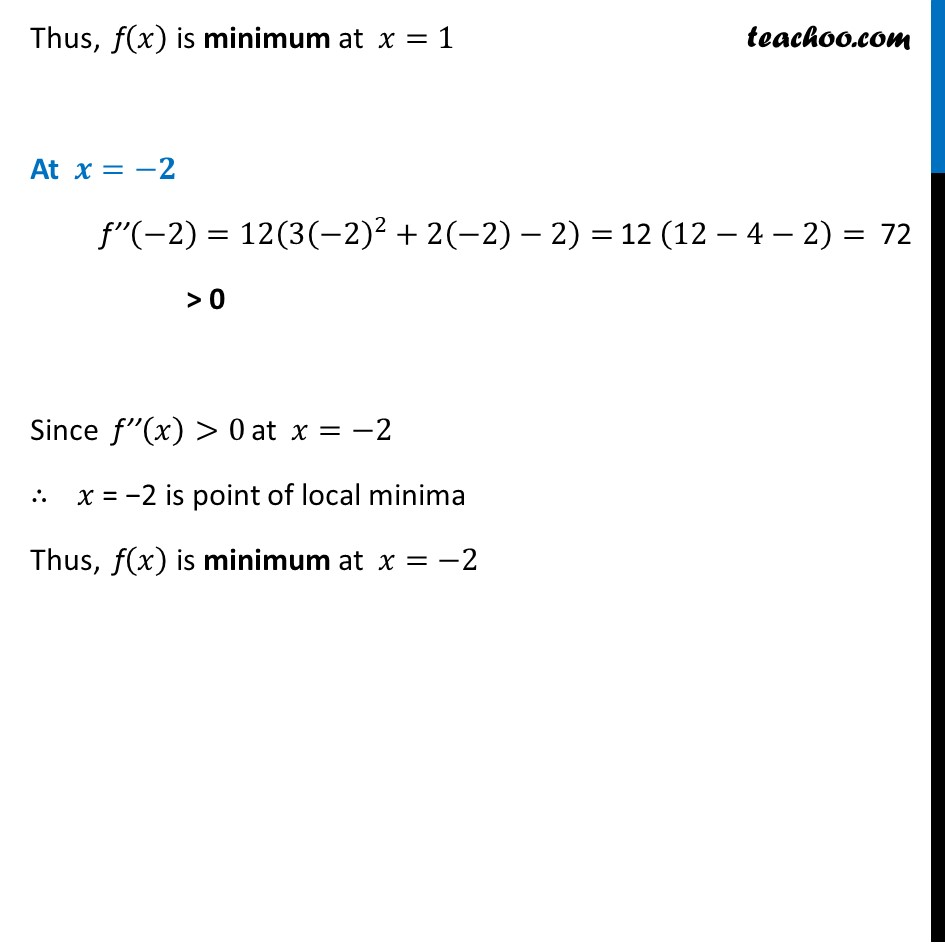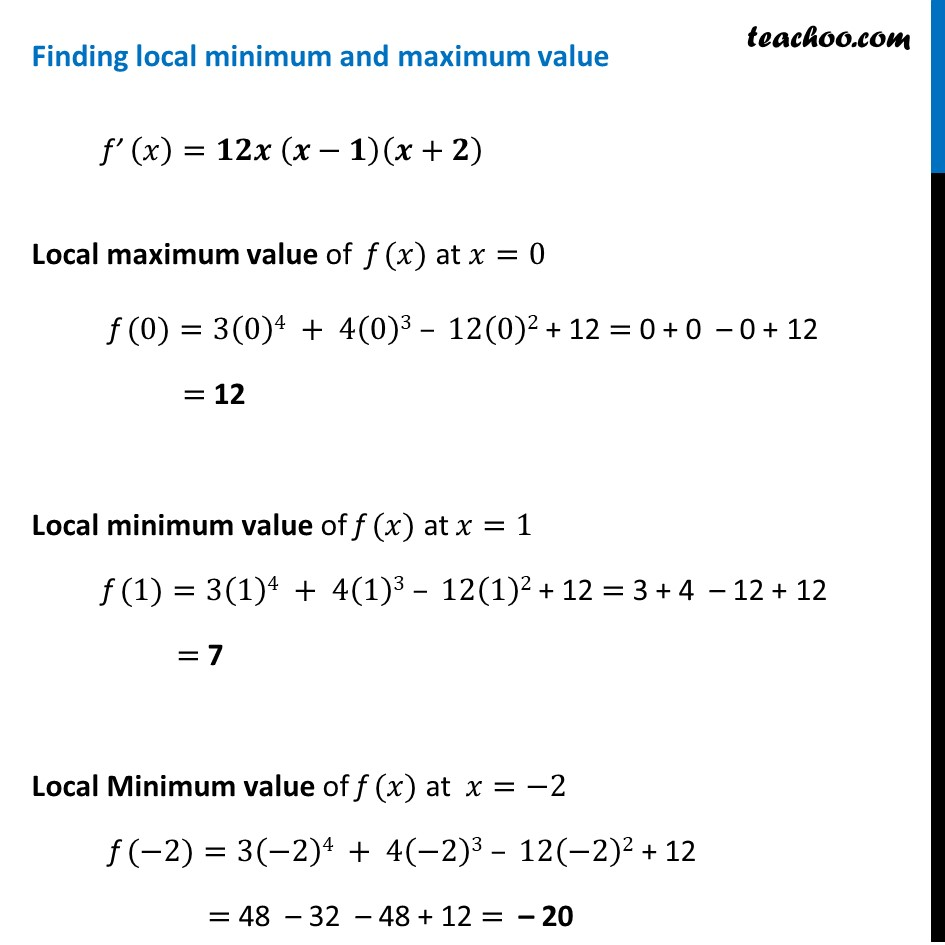Learn in your speed, with individual attention - Teachoo Maths 1-on-1 Class

### Transcript

Example 20 Find local maximum and local minimum values of the function f given by f (𝑥)=3𝑥4 + 4𝑥3 – 12𝑥2 + 12f (𝑥)=3𝑥4 + 4𝑥3 – 12𝑥2 + 12 Finding f’ (𝒙) f’ (𝑥)=𝑑(3𝑥4 + 4𝑥3 – 12𝑥2 + 12)/𝑑𝑥 f’ (𝑥)=12𝑥^3+12𝑥^2 – 24𝑥 "+ 0" f’ (𝑥)=12(𝑥^3+𝑥^2−2𝑥) f’ (𝑥)=12𝑥(𝑥^2+𝑥−2) f’ (𝑥)=12𝑥 (𝑥^2+2𝑥−𝑥−2) f’ (𝑥)=12𝑥 (𝑥(𝑥+2)−1(𝑥+2)) f’ (𝑥)=𝟏𝟐𝒙 (𝒙−𝟏)(𝒙+𝟐) Putting f’ (𝒙)=𝟎 12𝑥 (𝑥−1)(𝑥+2)=0 𝑥 (𝑥−1)(𝑥+2)=0 So, 𝒙=𝟎,𝑥=𝟏,& 𝑥=−𝟏 Finding f’’(𝒙) f ’(𝑥)=12(𝑥^3+𝑥^2−2𝑥) f ’’(𝑥)=12𝑑(𝑥^3 + 𝑥^2 − 2𝑥)/𝑑𝑥 f ’’(𝑥)=𝟏𝟐(𝟑𝒙^𝟐+𝟐𝒙−𝟐) At 𝒙=𝟎 f ’’(0)=12(3(0)^2+2(0)−2)= 32 (0+0 −2)= – 64 < 0 Since f’’(𝑥)<0 at 𝑥=0 ∴ 𝑥 = 0 is point of local maxima Thus, f(𝑥) is maximum at 𝑥=0 At 𝒙=𝟏 f’’(1)=12(3(1)^2+2(1)−2)= 12 (3+2−2) = 36 > 0 Since f’’(𝑥)>0 at 𝑥=1 ∴ 𝑥 = 1 is point of local minima Thus, f(𝑥) is minimum at 𝑥=1 At 𝒙=−𝟐 f’’(−2)=12(3(−2)^2+2(−2)−2)= 12 (12−4−2)= 72 > 0 Since f’’(𝑥)>0 at 𝑥=−2 ∴ 𝑥 = −2 is point of local minima Thus, f(𝑥) is minimum at 𝑥=−2 Finding local minimum and maximum value f’ (𝑥)=𝟏𝟐𝒙 (𝒙−𝟏)(𝒙+𝟐) Local maximum value of f (𝑥) at 𝑥=0 f (0)=3(0)4 + 4(0)3 – 12(0)2 + 12 = 0 + 0 – 0 + 12 = 12 Local minimum value of f (𝑥) at 𝑥=1 f (1)=3(1)4 + 4(1)3 – 12(1)2 + 12 = 3 + 4 – 12 + 12 = 7 Local Minimum value of f (𝑥) at 𝑥=−2 f (−2)=3(−2)4 + 4(−2)3 – 12(−2)2 + 12 = 48 – 32 – 48 + 12 = – 20